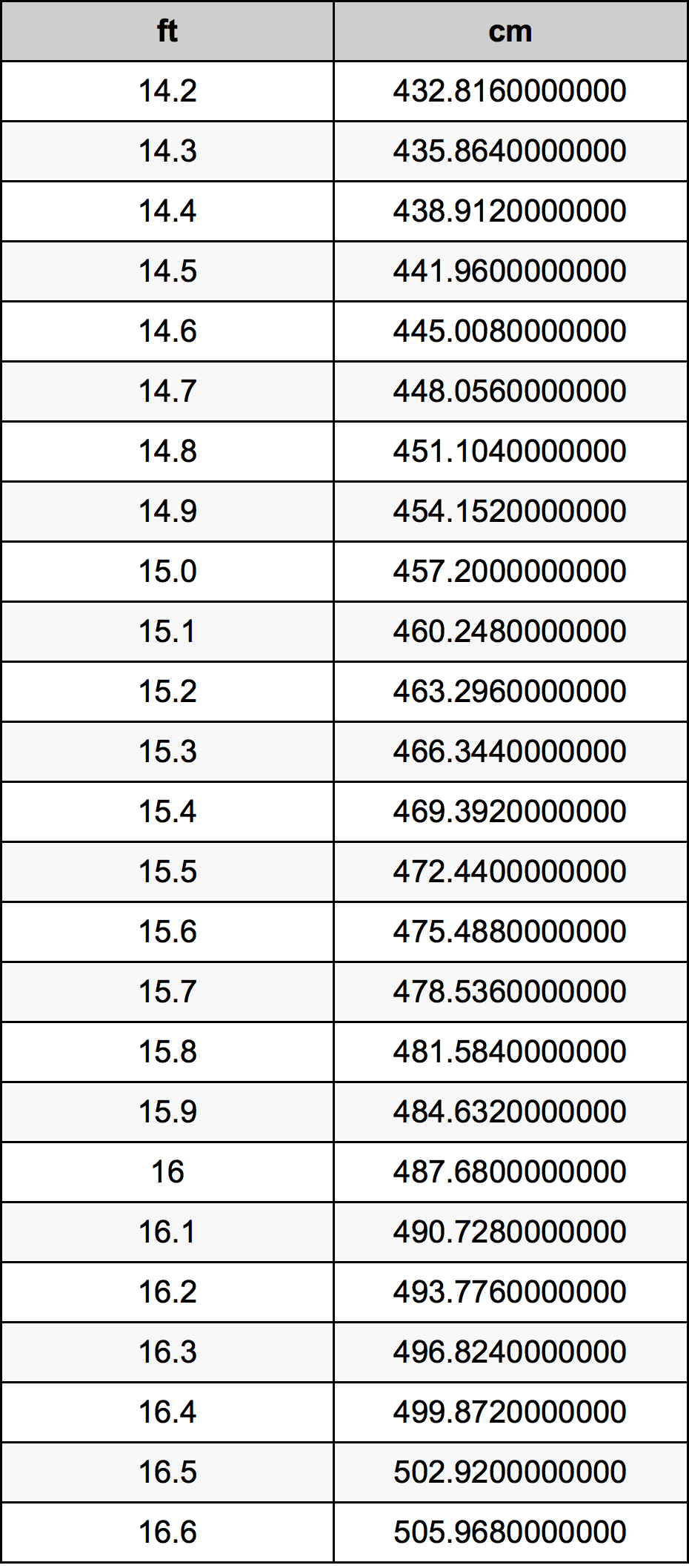Feet To Cm

# 15.4 ft to cm15.4 Feet to Centimeters

ft
=
cm

## How to convert 15.4 feet to centimeters?

 15.4 ft * 30.48 cm = 469.392 cm 1 ft
A common question is How many foot in 15.4 centimeter? And the answer is 0.5052493438 ft in 15.4 cm. Likewise the question how many centimeter in 15.4 foot has the answer of 469.392 cm in 15.4 ft.

## How much are 15.4 feet in centimeters?

15.4 feet equal 469.392 centimeters (15.4ft = 469.392cm). Converting 15.4 ft to cm is easy. Simply use our calculator above, or apply the formula to change the length 15.4 ft to cm.

## Convert 15.4 ft to common lengths

UnitUnit of length
Nanometer4693920000.0 nm
Micrometer4693920.0 µm
Millimeter4693.92 mm
Centimeter469.392 cm
Inch184.8 in
Foot15.4 ft
Yard5.1333333333 yd
Meter4.69392 m
Kilometer0.00469392 km
Mile0.0029166667 mi
Nautical mile0.002534514 nmi

## What is 15.4 feet in cm?

To convert 15.4 ft to cm multiply the length in feet by 30.48. The 15.4 ft in cm formula is [cm] = 15.4 * 30.48. Thus, for 15.4 feet in centimeter we get 469.392 cm.

## 15.4 Foot Conversion Table## Alternative spelling

15.4 Foot to Centimeters, 15.4 Foot in Centimeters, 15.4 Feet to cm, 15.4 Feet in cm, 15.4 Foot to Centimeter, 15.4 Foot in Centimeter, 15.4 ft to Centimeter, 15.4 ft in Centimeter, 15.4 Feet to Centimeters, 15.4 Feet in Centimeters, 15.4 Foot to cm, 15.4 Foot in cm, 15.4 ft to Centimeters, 15.4 ft in Centimeters